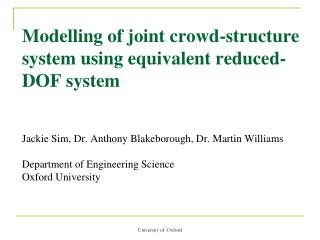DownloadDownload PresentationCantilever grandstands

# Cantilever grandstands

Download Presentation## Cantilever grandstands

- - - - - - - - - - - - - - - - - - - - - - - - - - - E N D - - - - - - - - - - - - - - - - - - - - - - - - - - -
##### Presentation Transcript

1. Modelling of joint crowd-structure system using equivalent reduced-DOF systemJackie Sim, Dr. Anthony Blakeborough, Dr. Martin WilliamsDepartment of Engineering ScienceOxford University University of Oxford

2. Cantilever grandstands University of Oxford

3. Dynamic analysis of cantilever grandstand Human-structure interaction Active crowd Load model Passive crowd Crowd model University of Oxford

4. Total mass of crowd = g ms ms ms F F x x Full model Crowd as 2DOF system Structure as SDOF system University of Oxford

5. ms F x Equivalent reduced DOF systems Equivalent SDOF system Equivalent 2DOF system Full model University of Oxford

6. Contents • Crowd model • Response of full model • Equivalent SDOF model • Equivalent 2DOF model University of Oxford

7. y2 DOF 2 m2 y2 m2 DOF 2 k2 c2 k2 c2 y1 DOF 1 m1 y1 k1 m1 c1 DOF 1 k1 c1 F m0 F Individual models – Griffin et al. Standing model Seated model University of Oxford

8. Crowd response University of Oxford

9. Crowd model Transfer functions: Seated: Standing: Fourth order polynomial i.e. 2DOF system University of Oxford

10. Crowd mass = g ms ms F x Dynamic analysis (1) 2% structural damping, Natural frequency of 1 to 10 Hz. 50% seated and 50% standing crowds g = 0%, 5%, 10%, 20%, 30% and 40% University of Oxford

11. Dynamic analysis (2) DMF = Peak displacement / Static displacement SDOF structure Displacement Excitation force Acceleration + Interaction force Seated / standing crowd University of Oxford

12. Results – DMF vs Frequency 2 Hz structure 4 Hz structure University of Oxford

13. Summary of results (1): Resonant frequency reduction factor F.R.F. = Change in frequency / Frequency of bare structure University of Oxford

14. Summary of results (2): DMF reduction factor DMF R.F. = Change in DMFmax / DMFmax of bare structure University of Oxford

15. Why reduced-DOF system? • Full crowd-model: 2DOF crowd + SDOF structure • A simplified model for • Easier analysis • Insight into the dynamics University of Oxford

16. Equivalent SDOF system • SDOF system transfer function: • Curve-fit DMF frequency response curve over bandwidth University of Oxford

17. Dynamic properties University of Oxford

18. Peak DMF relative error Error analysis (1) Resonant frequency relative error University of Oxford

19. Error analysis (2) University of Oxford

20. ms F x Equivalent 2DOF system Crowd modelled as SDOF system Structure remains the same SDOF system University of Oxford

21. SDOF crowd model University of Oxford

22. Dynamic analysis SDOF structure Displacement Excitation force Acceleration + Interaction force SDOF Seated / standing crowd University of Oxford

23. Error analysis University of Oxford

24. Bode diagrams University of Oxford

25. Conclusions • Passive crowd adds significant damping • 1 to 4 Hz – behaviour of a SDOF system • > 4 Hz – behaviour of a 2DOF system University of Oxford